# The carpenters

The carpenters cut the beam. Half of the cut pieces were 1 meter long, a quarter of the pieces were two meters long and the remaining 8 meters of the beam were cut into two equal parts.
- What was the length of the original beam?
- What part of the original beam was cut into meter pieces?

a =  16 m
b =  0.25

### Step-by-step explanation:

$b={d}_{1}\mathrm{/}a=4\mathrm{/}16=\frac{1}{4}=0.25$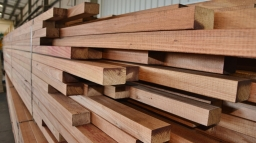Did you find an error or inaccuracy? Feel free to write us. Thank you!Tips to related online calculators
Need help to calculate sum, simplify or multiply fractions? Try our fraction calculator.
Do you have a linear equation or system of equations and looking for its solution? Or do you have a quadratic equation?
Do you want to convert length units?

## Related math problems and questions:

• Wiring 2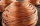Willie cut a piece of wire that was 3/8 of the total length of the wire. He cut another piece that was 9m long. The two pieces together were one-half of the total length of the wire. How long was the wire before he cut it?
• Half of halvesHalf of the square we cut off, then half of the rest, etc. Five cuts we made in this way. What part of the content of the original square is the content of the cut part?
• SeamstressThe seamstress cut the fabric into 3 parts. The first part was the eighth fabric, the second part was three-fifths of the fabric, and the third part had a length of 66 cm. Calculate the original length of the fabric.
• Prices pricesThe meter of the textille was reduced by CZK 42, so 4 meters of the new price was 20 CZK cheaper than 3 meters of the original price. What was the original and new price of the textille?
• StoreOne meter of the textile was discounted by 2 USD. Now 9 m of textile cost as before 8 m. Calculate the old and new price of 1 m of the textile.
• Hexagon = 8 parts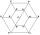Divide the regular hexagon into eight equal parts.
• Carpenter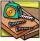You want to cut long board into smaller pieces. Carpenter said that one cut of the board into two pieces will cost you 70 cents. But you need to cut the board into 9 parts. How much you pay?
• Wire cut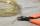A wire of length 9 m was cut into equal lengths using 5 cuts. How long is each piece?
• Wood 11Father has 12 1/5 meters long wood. Then I cut the wood into two pieces. One part is 7 3/5 meters long. Calculate the length of the other wood?
• A PVC pipeA PVC pipe 12 feet long is to be cut into 1 1/2 foot pieces.  How many pieces can a plumber get out of this length pipe?
• DiscountThe dress was discounted by CZK 115, which was 12% of the original price. What is the cost of the dress after the discount?
• Krkonose CZ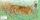Tourist's rod on the tourist route in the Krkonose was 1/5 of its length into the ground. Snow fell in winter and 1/3 of the length of the rod remained above the snow. Find the height of the snow if the length of the part above the snow is 32 cm greater t
• Two numbersThe sum of the two numbers is 1. Find both numbers if you know that half of the first is equal to one seventh of the second number.
• Pet store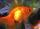In a pet store, they are selling out the fish from one aquarium. Ondra wanted half of all fish, but they don't wish cut by hal fany fish he got one more than demanded. Matthew wished the remaining half of the fish, but as Andrew got half the fish more tha
• The vase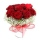There were roses in the vase, half were red, a quarter was white and 12 were yellow. How many roses were in the vase?
• The mowers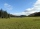The mowers were to mow two meadows, one twice as big as the other. In the first half of the day, they divided into two equal groups. One continued to mow a larger meadow and cut it all by the end of the day. The second group mowed a smaller meadow but did
• Copper partsFrom copper cast were made 3 parts. At first consumed one third of casting, the second 2/3 of rest and the third weighed 18 kg. What is the weight of original copper cast?